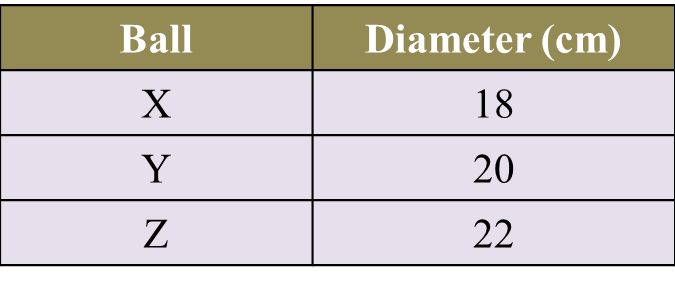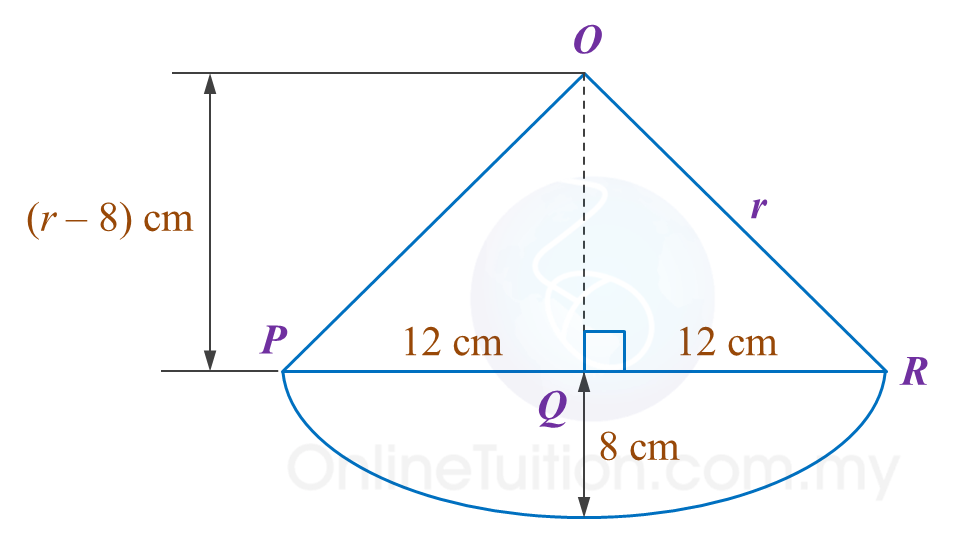# 10.2.3 Circles I, PT3 Focus Practice

            
Question 11:
  
The Town Council plans to build an equilateral triangle platform in the middle of a roundabout. The diameter of circle RST is 24 m and the perpendicular distance from R to the line ST is 18 m. as shown in Diagram below.Find the perimeter of the platform.
  
  Solution:Given diameter = 24 m
  hence radius = 12 m
  O is the centre of the circle.
  Using Pythagoras’ theorem:
            
  
 
      
Question 12:
 
Amy will place a ball on top of a pillar in Diagram below. Table below shows the diameters of three balls X, and Z.Which ball X, or Z, can fit perfectly on the top of the pillar? Show the calculation to support Amy’s choice.
 
 Solution:Question 13:
 
Diagram below shows a rim of a bicycle wheel with a diameter of 26 cm. Kenny intends to build a holder for the rim.Which of the rim holder, X, or Z, can fit the bicycle rim perfectly? Show the calculation to support your answer.
 
 Solution: